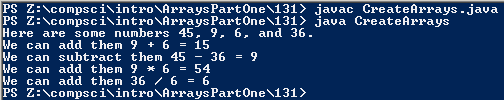# Assignemnt #131 and Create Arrays

## Code

```    ///Name: Daniel Tiffany-Appleton
///Period: 7
///Program Name: Create Arrays
///File Name: CreateArrays
///Date Finished: 4/28/16

public class CreateArrays
{
public static void main(String[] args)
{
int[] intArray = {45, 9, 6, 36};

System.out.println("Here are some numbers " + intArray + ", " + intArray + ", " + intArray + ", and " + intArray + ".");

int add = intArray + intArray;
int sub = intArray - intArray;
int multi = intArray * intArray;
int div = intArray / intArray;
System.out.println("We can add them " + intArray + " + " + intArray + " = " + add);
System.out.println("We can subtract them " + intArray + " - " + intArray + " = " + sub);
System.out.println("We can add them " + intArray + " * " + intArray + " = " + multi);
System.out.println("We can add them " + intArray + " / " + intArray + " = " + div);

}
}

```

### Picture of the output